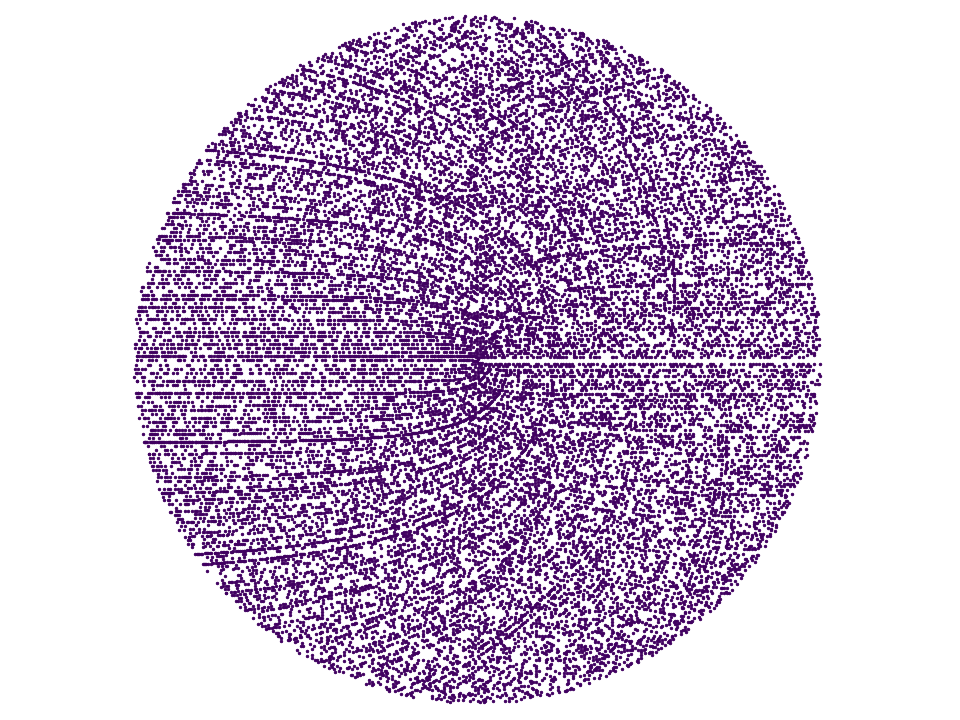# Archimedes Prime Spiral

Byon November 8, 2015

Keywords: Archimedes prime spiral

This was a fun little Sunday morning coding project I threw together in a little over an hour. Inspired by some prime number videos from numberphile I decided to throw together a simple program to generate an Archimedes prime number spiral.Figure [prime-spiral]. This is a prime number graph plotted on an Archimedes spiral showing the first 24,000 prime numbers.

Here is the code to do it, although it could stand some cleaning up. The function to test for primality is crude, but considering we are only dealing with prime numbers less than one million it isn't a big deal. Case in point, the program determines that the 24,000$$^{th}$$ prime number is 274,529 . On my computer it takes only about 12 seconds to finish.

// prime_spiral.c : generate prime spiral gnuplot file

#include <stdio.h>
#include <stdlib.h>
#include <complex.h>
#include <math.h>
#include <liquid/liquid.h>

#define OUTPUT_FILENAME "blog/prime-spiral/prime_spiral.gnu"

int main(int argc, char*argv[])
{
// parameters and simulation options
unsigned int max_primes = 24000;    // number of primes

// open output file
FILE * fid = fopen(OUTPUT_FILENAME,"w");
if (!fid) {
fprintf(stderr,"error: %s, could not open '%s' for writing\n", argv, OUTPUT_FILENAME);
return -1;
}
fprintf(fid,"# %s: auto-generated file\n\n", OUTPUT_FILENAME);
fprintf(fid,"reset\n");
fprintf(fid,"set term pngcairo size 960,720 enhanced font 'Verdana,10'\n");
fprintf(fid,"set size ratio 1.0\n");
fprintf(fid,"#set xlabel 'Sample Index'\n");
fprintf(fid,"#set key top right nobox\n");
fprintf(fid,"#set grid xtics ytics\n");
fprintf(fid,"#set grid linetype 1 linecolor rgb '#cccccc' lw 1\n");
fprintf(fid,"#set grid xtics ytics\n");
fprintf(fid,"\n");
fprintf(fid,"set pointsize 0.4\n");
fprintf(fid,"set nobox\n");
fprintf(fid,"unset xtics\n");
fprintf(fid,"unset ytics\n");
fprintf(fid,"unset colorbox\n");
fprintf(fid,"set border 0\n");
fprintf(fid,"set key off\n");
fprintf(fid,"plot '-' using 5:6 with points pt 7 linecolor rgb '#400060' title 'real'\n");

unsigned int num_primes = 0;    // number of primes found
unsigned int p          = 2;    // prime number candidate
unsigned int a          = 1;    // ring
unsigned int b          = a*a;  //
unsigned int b0         = (a-1)*(a-1);
while (num_primes < max_primes) {
// test for primality
if (liquid_is_prime(p)) {
num_primes++;
float r     = (float)a;
float theta = 2*M_PI*( (float)(p-b0)/(float)(b-b0) );
float x     = r * cosf(theta);
float y     = r * sinf(theta);
fprintf(fid,"  %8u %8u %8u %8u %12.4e %12.4e\n", num_primes, p, a, b, x, y);
}

// increment prime number candidate: 2,3,5,7,9,11,...
p += (p == 2) ? 1 : 2;

// increment roots
if (p > b) {
a++;
b0 = b;
b = a*a;
}
}
fprintf(fid,"e\n");

fclose(fid);
printf("results written to %s\n", OUTPUT_FILENAME);

return 0;
}